# HSSlive: Plus One & Plus Two Notes & Solutions for Kerala State Board

## AP Board Class 8 Maths Chapter 12 Factorisation Ex 12.2 Textbook Solutions PDF: Download Andhra Pradesh Board STD 8th Maths Chapter 12 Factorisation Ex 12.2 Book AnswersAP Board Class 8 Maths Chapter 12 Factorisation Ex 12.2 Textbook Solutions PDF: Download Andhra Pradesh Board STD 8th Maths Chapter 12 Factorisation Ex 12.2 Book Answers

## Andhra Pradesh State Board Class 8th Maths Chapter 12 Factorisation Ex 12.2 Books Solutions

 Board AP Board Materials Textbook Solutions/Guide Format DOC/PDF Class 8th Subject Maths Chapters Maths Chapter 12 Factorisation Ex 12.2 Provider Hsslive

2. Click on the Andhra Pradesh Board Class 8th Maths Chapter 12 Factorisation Ex 12.2 Answers.
3. Look for your Andhra Pradesh Board STD 8th Maths Chapter 12 Factorisation Ex 12.2 Textbooks PDF.

## AP Board Class 8th Maths Chapter 12 Factorisation Ex 12.2 Textbooks Solutions with Answer PDF Download

Find below the list of all AP Board Class 8th Maths Chapter 12 Factorisation Ex 12.2 Textbook Solutions for PDF’s for you to download and prepare for the upcoming exams:

Question 1.
Factorise the following expression
i) a2 + 10a +25
ii) l2 – 16l + 64
iii) 36x2 + 96xy + 64y2
iv) 25x2 + 9y2 – 30xy
v) 25m2– 40mn + 1 6n2
vi) 81x2 – 198 xy + 12ly2
vii) (x+y)2 – 4xy
(Hint : first expand ( x + y)2 )
viii) l4 + 4l2m2 + 4m4
Solution:
i) a2 + 10a +25
= (a)2 + 2 × a × 5 + (5)2
It is in the form of a2 + 2ab + b2
a2 + 2ab + b2= (a + b)2
∴ a2 + 10a + 25 = (a + 5)2 = (a + 5) (a + 5)

ii) l2 – 16l + 64
l2 – 16l + 64
= (l)2 – 2 × l × 8 + (8)2
It is in the form of a2 – 2ab + b2
a2 – 2ab + b2 = (a – b)2
∴ l2 – 16l + 64 = (l – 8)2 = (l – 8) (l – 8)

iii) 36x2 + 96xy + 64y2
36x2 + 96xy + 64y2
= (6x)2 + 2 × 6x × 8y + (8y)2
It is in the form of a2 + 2ab + b2
a2 + 2ab + b2 = (a + b)2
∴ 36x2 + 96xy + 64y2
= (6x + 8y)2 = (6x + 8y) (6x + 8y)

iv) 25x2 + 9y2 – 30xy
25x2 + 9y2 – 30xy
= (5x)2 + (3y)2 – 2 × 5x × 3y
It is in the form of a2 + b2 – 2ab
a2 + b2 – 2ab = (a – b)2
∴ 25x2 + 9y2 – 30xy
= (5x – 3y)2 = (5x – 3y) (5x – 3y)

v) 25m2– 40mn + 1 6n2
25m2 – 40mn + 16n2
= (5m)2 – 2 × 5m × 4n + (4n)2
It is in the form of a2 – 2ab + b2
a2 – 2ab + b2 = (a – b)2
∴ 25m2 – 40mn + 16n2
= (5m – 4n)2
= (5m – 4n) (5m – 4n)

vi) 81x2 – 198 xy + 12ly2
81x2 – 198xy + 121y2
= (9x)2 – 2 × 9x × 11y + (11y)2
It is in the form of a2 – 2ab + b2
a2 – 2ab + b2 = (a – b)2
∴ 81x2 – 198xy + 121y2
= (9x – 11y)2 – (9x – 11y) (9x – 11y)

vii) (x+y)2 – 4xy
(Hint : first expand ( x + y)2 )
= (x + y)2 – 4xy
= x2 + y2 + 2xy – 4xy
= x2 + y2 – 2xy = (x – y)2 = (x – y)(x – y)

viii) l4 + 4l2m2 + 4m4
l4 + 4l2m2 + 4m4
= (l2)2 + 2 × l2 × 2m2 + (2m2)2
It is in the form of a2 + 2ab + b2
a2 + 2ab + b2 = (a – b)2
∴ l4 + 4l2m2 + 4m4
= (l2 + 2m2)2 = (l2 + 2m2) (l2 + 2m2)

Question 2.
Factorise the following
i) x2 – 36
ii) 49x2 – 25y2
iii) m2 – 121
iv) 81 – 64x2
v) x2y2 – 64
vi) 6x2 – 54
vii) x2 – 81
viii) 2x -32 x5
ix) 81x4 – 121x2
x) (p2 – 2pq + q2)-r2
xi) (x+y)2 – (x-y)2
Solution:
i) x2 – 36
x2 – 36
⇒ (x)2 – (6)2 is in the form of a2 – b2
a2 – b2 = (a + b) (a – b)
∴ x2 – 36 = (x + 6) (x – 6)

ii) 49x2 – 25y2
= (7x)2 – (5y)2
= (7x + 5y) (7x – 5y)

iii) m2 – 121
m2 -121
= (m)2 – (11)2
= (m + 11) (m – 11)

iv) 81 – 64x2
81 – 64x2
= (9)2 – (8x)2
= (9 + 8x) (9 – 8x)

v) x2y2 – 64
= (xy)2 – (8)2
= (xy + 8)(xy – 8)

vi) 6x2 – 54
6x2 – 54
= 6x2 – 6 x 9 ‘
= 6(x2 – 9)
= 6[(x)2 – (3)2]
= 6(x + 3) (x – 3)

vii) x2 – 81
x2 – 81
= x2 – 92
= (x + 9 )(x – 9)

viii) 2x – 32 x5
2x – 32 x5
= 2x – 2x x 16x4
= 2 x (1 – 16x4)
= 2x [12) – (4x2)2]
= 2x (1 + 4x2) (1 – 4x2)
= 2x (1 + 4x2) [(15 – (2x)2]
= 2x (1 + 4x2) (1 + 2x) (1 – 2x)

ix) 81x4 – 121x2
81x4 – 121x2
– x2 (812 – 121)
= x2[(9x)2 – (11)2]
= x2 (9x + 11) (9x -11)

x) (p2 – 2pq + q2)-r2
(p2 – 2pq + q2) – r2
= (p – q)2 – (r)2 [∵ p2 – 2pq + q2 = (p – q)2]
= (p – q + r) (p – q – r)

xi) (x + y)2 – (x – y)2
(x + y)2 – (x – y)2
It is in the form of a2 – b2
a = x + y, b = x- y
∴ a2 – b2 =(a + b)(a-b)
= (x + y + x – y) [x + y- (x – y)]
= 2x [x + y-x + y]
= 2x x 2y = 4xy

Question 3.
Factorise the expressions
(i) lx2 + mx
(ii) 7y2 + 35Z2
(iii) 3x4 + 6x3y + 9x2Z
(iv) x2 – ax – bx + ab
(v) 3ax – 6ay – 8by + 4bx
(vi) mn + m + n + 1
(vii) 6ab – b2 + 12ac – 2bc
(viii) p2q – pr2 – pq + r2
(ix) x (y + z) -5 (y + z)

(i) lx2 + mx
lx2 + mx
= l × x × x + m × x = x(lx + m)

(ii) 7y2 + 35z2
7y2+ 35z2
= 7 × y2 + 7 × 5 × z2
= 7(y2 + 5z2)

(iii) 3x4 + 6x3y + 9x2Z
3x4 + 6x3y + 9x2Z
= 3 × x2 × x2 + 3 × 2 × x × x2 × y + 3 × 3 × x2 × z
= 3x2 (x2 + 2xy + 3z)

(iv) x2 – ax – bx + ab
x2 – ax – bx + ab
= (x2 – ax) – (bx – ab)
= x(x – a) – b(x – a)
= (x – a) (x – b)

(v) 3ax – 6ay – 8by + 4bx
3ax – 6ay – 8by + 4bx
= (3ax – 6ay) + (4bx – 8by)
= 3a (x – 2y) + 4b (x – 2y)
= (x – 2y) (3a + 4b)

(vi) mn + m + n + 1
mn + m + n + 1
= (mn + m) + (n + 1)
= m (n + 1) + (n + 1)
= (n + 1) (m + 1)

(vii) 6ab – b2 + 12ac – 2bc
6ab – b2 + 12ac – 2bc
= (6ab – b2) + (12ac – 2bc)
= (6 × a× b – b × b) + (6 × 2 × a × c – 2 × b × c)
= b [6a – b] + 2c [6a – b]
= (6a – b) (b + 2c)

(viii) p2q – pr2 – pq + r2
p2q – pr2 – pq + r2
= (p2q – pr2) – (pq – r2)
= (p × p × q – p × r × r) – (pq – r2)
= P(pq – r2) – (pq – r2) × 1
= (pq – r2)(p – 1)

(ix) x (y + z) -5 (y + z)
= x(y + z) – 5(y + z)
= (y + z) (x – 5)

Question 4.
Factorise the following
(i) x4 – y4
(ii) a4 – (b + c)4
(iii) l2 – (m – n)2
(iv) 49x2 – 1625
(v) x4 – 2x2y2 + y4
(vi) 4 (a + b)2 – 9 (a – b)2
Solution:
= (x2)2 – (y2)2 is in the form of a2 – b2
a2 – b2 = (a + b) (a – b)
x4 – y4 = (x2 + y2)(x2 – y2)
= (x2 + y2)(x + y)(x – y)

(ii) a4 – (b + c)4
a4 – (b + c)4
= (a2)2 – [(b + c)2]2
= [a2 + (b + c)2] [a2 – (b + c)2] ,
= [a2 + (b + c)2] (a + b + c) [a – (b + c)]
= [a2 + (b + c)2] (a + b + c) (a – b – c)

(iii) l2 – (m – n)2
l2 – (m – n)2
= (l)2 – (m – n)2
= [l + m – n] [l – (m – n)]
= [l + m -n] [l – m + n]

(iv) 49x2 – 1625
= (7x)2 – (45)2
= (7x+ (45) (7x – (45)

(v) x4 – 2x2 y2 + y4
= (x2 )2 – 2x2 y2 + (y2 )2
It is in the form of a2 – 2ab + b2
a2 – 2ab + b2 = (a – b)2
∴ x4 – 2x2 y2 + y4 = (x2 – y2 )2
= [(x)2 – (y)2 ]2
= [(x + y) (x – y)]2
= (x + y)2 (x – y)2
[∵ (ab)m = a m . bn ]

(vi) 4 (a + b)2 – 9 (a – b)2
4 (a + b)2 – 9 (a – b)2
= [2(a + b)]2 – [3(a – b)]2
= [2(a + b) + 3(a- b)] [2(a + b)-3(a- b)]
= (2a + 2b + 3a – 3b) (2a + 2b – 3a + 3b)
= (5a – b) (5b – a)

Question 5.
Factorise the following expressions
(i) a2+ 10a + 24
(ii) x2 +9x + 18
(iii) p2 – 10q + 21
(iv) x2 – 4x – 32
Solution:
(i) a2+ 10a + 24
a2 + 10a + 24 .
= a2 + 6a + 4a + 24
= a x a + 6a + 4a + 6 × 4
= a(a + 6) + 4(a + 6)
= (a + 6) (a + 4) (or)
a2 + 10a + 24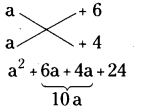∴ a2 + 10a + 24 = (a + 6) (a + 4)

(ii) x2 + 9x + 18
x2 + 9x + 18
= (x + 3) (x + 6)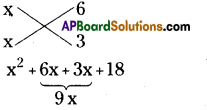∴ x2 + 9x + 18 = (x + 3) (x + 6)

(iii) p2 – 10q + 21
p2 – 10p + 21
= (P – 7) (p – 3)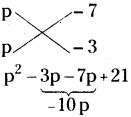∴ p2 – 10p + 21 = (p – 7)(p – 3)

(iv) x2 – 4x – 32
x2 – 4x – 32
= (x – 8) (x + 4)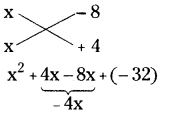∴ x2 – 4x – 32 = (x – 8) (x + 4)

Question 6.
The lengths of the sides of a triangle are integrals, and its area is also integer. One side is 21 and the perimeter is 48. Find the shortest side.
Solution:
Perimeter of a triangle
= AB + BC + CA = 48
⇒ c + a + b = 48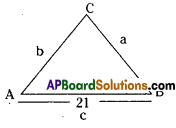The solutions of Harmeet, Rosy are wrong.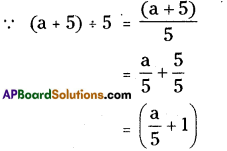∴ Srikar had done it correctly.
⇒ 21 + a + b = 48
⇒ a + b = 48 – 21 = 27
∴ The lengths of a, b should be 10, 17
∴ a + b > c [the sum of any two sides of a triangle is greater than the 3rd side]
∴ 10 + 17 > 2
27 > 21 (T).
∴ The length of the shortest side is 10 cm.

Question 7.
Find the values of ‘m’ for which x2 + 3xy + x + my – in has two linear factors in x and y, with integer coefficients.
Solution:
Given equation is x2 + 3xy + x + my – m ……….(1)
Let the two linear equations in x and y be (x + 3y + a) and (x + 0y + b).
Then (x + 3y + a) (x + 0y + b)
= x2 + 0xy + bx + 3xy + 0y2 + 3by + ax + 0y + ab
= x2 + bx + ax + 3xy + 3by + ab ………….. (2)
Comparing equation (2) with (1),
x2 + 3xy + x + my – m
= x2 + (a + b)x + 3xy + 3by + ab
Equating the like terms on both sides,
ab = – m ………….. (3)
(a + b)x = x ⇒ a + b = 1 ……………. (4)
3by = my ⇒ 3b = m ⇒ b = m3
Substitute ‘b’ value in equation (4),
a = 1−𝑚3=3−𝑚3
ab = -m
[ ∵ from (3)]
put a & b value then ,
(3−𝑚3)(𝑚3) = -m
3 m−m29= -m
⇒ 3m – m2 = – 9m
⇒ m2 – 12m = 0
⇒ m(m – 12) = 0
⇒ m = 0 (or) m = 12
lf m = 12

∴ b = 123 = 4&a = 3−m3=3−123
= −93 = -3
∴ Linear factors are (x + 3y – 3), (x + 4) If m = 0
b = 03 = 0 & a = 3−03=33 = 1
∴ Linear factors are (x + 3y + 1), x.

## Andhra Pradesh Board Class 8th Maths Chapter 12 Factorisation Ex 12.2 Textbooks for Exam Preparations

Andhra Pradesh Board Class 8th Maths Chapter 12 Factorisation Ex 12.2 Textbook Solutions can be of great help in your Andhra Pradesh Board Class 8th Maths Chapter 12 Factorisation Ex 12.2 exam preparation. The AP Board STD 8th Maths Chapter 12 Factorisation Ex 12.2 Textbooks study material, used with the English medium textbooks, can help you complete the entire Class 8th Maths Chapter 12 Factorisation Ex 12.2 Books State Board syllabus with maximum efficiency.

## FAQs Regarding Andhra Pradesh Board Class 8th Maths Chapter 12 Factorisation Ex 12.2 Textbook Solutions

#### Can we get a Andhra Pradesh State Board Book PDF for all Classes?

Yes you can get Andhra Pradesh Board Text Book PDF for all classes using the links provided in the above article.

## Important Terms

Andhra Pradesh Board Class 8th Maths Chapter 12 Factorisation Ex 12.2, AP Board Class 8th Maths Chapter 12 Factorisation Ex 12.2 Textbooks, Andhra Pradesh State Board Class 8th Maths Chapter 12 Factorisation Ex 12.2, Andhra Pradesh State Board Class 8th Maths Chapter 12 Factorisation Ex 12.2 Textbook solutions, AP Board Class 8th Maths Chapter 12 Factorisation Ex 12.2 Textbooks Solutions, Andhra Pradesh Board STD 8th Maths Chapter 12 Factorisation Ex 12.2, AP Board STD 8th Maths Chapter 12 Factorisation Ex 12.2 Textbooks, Andhra Pradesh State Board STD 8th Maths Chapter 12 Factorisation Ex 12.2, Andhra Pradesh State Board STD 8th Maths Chapter 12 Factorisation Ex 12.2 Textbook solutions, AP Board STD 8th Maths Chapter 12 Factorisation Ex 12.2 Textbooks Solutions,
Share: